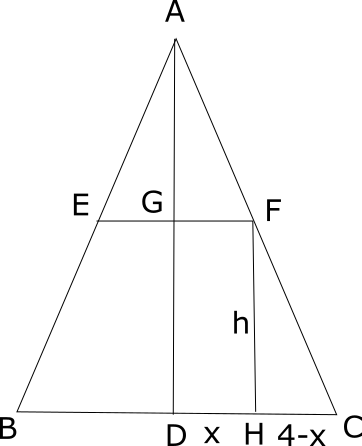Question 25

# Consider a right circular cone of base radius 4 cm and height 10 cm. A cylinder is to be placed inside the cone with one of the flat surfaces resting on the base of the cone. Find the largest possible total surface area (in sq. cm) of the cylinder.

SolutionTrangle AGF and triangle FHC are similar.
So, $$\frac{10-h}{x}=\frac{h}{4-x}$$
=> $$\frac{10-h}{h}=\frac{x}{4-x}$$
=> $$\frac{10}{h}-1=\frac{x}{4-x}$$
=> $$\frac{10}{h}=\frac{4}{4-x}$$
=> $$h=\frac{10}{4}(4-x)$$

Total surface area= $$2 \pi x^2 + 2 \pi x h= 2 \pi (x^2 + x \frac{10}{4}(4-x)=2 \pi (10x - \frac{3}{2} (x^2))$$

=$$2\pi\frac{-3}{2}[x^{2}-\frac{20}{3}x]$$. We can add and subtract 100/9 to get a perfect square.

=$$2\pi\frac{-3}{2}[x^{2}-\frac{20}{3}x+\frac{100}{9}-\frac{100}{9}]$$

=$$2 \pi (-\frac{3}{2}(x-\frac{10}{3})^{2}+ \frac{50}{3})$$

This value will be maximum when the negative factor is minimum i.e. 0 because it is multiplied by a square. Thus, when x=10/3 the surface area is max.

So, the surface area is $$\frac{100}{3} \pi$$